Next: Minimizing the Huber function Up: Algorithm for minimizing the Previous: The limited memory BFGS

# An efficient algorithm for solving nonlinear problems

The solver works as follows:
1.
Choose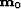, l,. Set k=0.
2.
Compute(79) (80)
where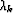meets the Wolfe conditions.
3.
Let=min,. Update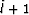times using the pairs, i.e., let(81) (82)
4.
Set k=k+1 and go to 2 if the residual power is not small enough.
The updateis not formed explicitly; instead we computewith an iterative formula Nocedal (1980). Liu and Nocedal (1989) propose scaling the initial symmetric positive definiteat each iteration as follows: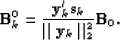(83)
This scaling greatly improves the performances of the method. Liu and Nocedal (1989) show that the storage limit for large-scale problems has little effects. A common choice for l is l=5. In practice, the initial guessfor the Hessian is the identity matrix; then it might be scaled as proposed in equation (). The nonlinear solver as detailed in the previous algorithm converges to a local minimizerof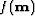.Next: Minimizing the Huber function Up: Algorithm for minimizing the Previous: The limited memory BFGS
Stanford Exploration Project
5/5/2005